# Analyze Causal Effect Using Diff-in-Diff Model

## With a real-world application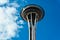Image by Author

It is not always feasible to do randomized AB experiments, but we can still recover the causal effect of a treatment if we have a near-experiment that generates observational data over time (i.e. panel data). One of the models we can use to estimate causal effect with observational data is Difference-in-Difference (Diff-in-Diff, or DiD).

# Diff-in-Diff Model

A Diff-in-Diff model applies when we have two existing groups (e.g. two regions A and B) not randomly assigned by us as in a randomized AB trial and a treatment happens to one of the groups (e.g. only region A launches a sales promotion), we can…

# Analyze the Effect of Randomized Experiment using the Tennessee STAR Experiment

## Analyze the causal effect of class size using linear regression in Python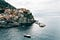Photo by Shuangyuan Wei on Unsplash

Randomized experiment or randomized control trial (RCT) is regarded as the gold standard to test causality. In the tech industry, RCT takes the form of online platform experiments and is often called A/B testing.

In this article, I will walk through the potential outcome model underlying the inference of the causal effect from an RCT, and replicate the econometric analysis of the Tennessee STAR experiment by Krueger (1999) in Python.

# Potential Outcome Model

An RCT takes a group of subjects and randomly assigns them to either a treatment group, which gets the policy treatment/intervention or a control group, which receives no treatment. In…

# Testing the Assumptions of Linear Regression

## Build a linear regression model step by step to test the Gauss-Markov assumptions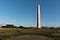Image by Author

It seems that nowadays when everyone is so much into all kinds of fancy machine learning algorithms, few people still care to ask: what are the key assumptions required for the Ordinary Least Squares (OLS) regression? How can I test if my model satisfies these assumptions? However, as simple linear regression is arguably the most popular modeling approach across every field in social science, I think it is worthwhile to do a quick recap of the fundamental assumptions for OLS and run some tests through building a linear regression model using the classic Boston Housing data.

# 1. Gauss-Markov Assumptions

The Gauss-Markov assumptions assure…

# An Illustration of Survey Sampling and Weighting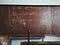Photo by Roman Mager on Unsplash

Survey is widely used in social science, public opinion polling, and marketing research. I use survey when the data I need to answer my questions can not be found in any existing data tables or be scraped from some webpages, so I have to go ask for the data myself. Survey is an important tool to generate original data. On the surface level, survey appears to be fairly easy to do — anyone can run a survey as long as they have a list of contact information and a questionnaire. …

# Randomized Experiment and Potential Outcome Model

## Dive deep into the importance of randomization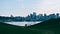Photo by Shuangyuan Wei on Unsplash

Randomized experiment or randomized control trial (RCT) is regarded as the gold standard to test causality in medicine, science, and social science. In the tech industry, RCT takes the form of an online platform experiment and is often called A/B testing.

An RCT takes a group of subjects and randomly assigns them to either a treatment group, which gets the policy treatment/intervention or a control group, which receives no treatment. in an RCT, the subjects are assigned to treatment and control by the flip of a coin. …

# Use Input-Output Model to Assess Economic Impact

## How to perform an economic impact analysis step by step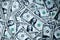Photo by Sharon McCutcheon on Unsplash

In this article, I will introduce the Input-Output model framework, explain the structure of an input-output table, and walk through step-by-step how to perform an economic impact analysis.

# Introduction

The Input-Output model is a framework developed by Dr. Leontief, in recognition of which he received the Nobel Prize in Economic Science, to analyze the interdependencies between different industries in an economy. The model is widely recognized and used by developers, urban planners, and government officials to assess the potential economic impacts of various projects.

The intuition behind behind the Input-Output model is that an initial change in economic activity results would…

# Understanding Logistic Regression using Log Odds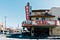Photo by Shuangyuan Wei on Unsplash

I had to adjust my thinking when it comes to logistic regression because it models a probability rather than a mean and it involves the non-linear transformation. In this article, I will explain the log odds interpretation of logistical regression in math, and also run a simple logistical regression model with real data.

The odds of an event are the probability of an event that it happens over the probability that it doesn’t. For example, if the P (success) = 0.8, and P (failure) = 0.2, the odds of success will be 0.8/0.2=4.

We use logistic regression to model a…## Shuangyuan (Sharon) Wei

data scientist, rock climber